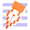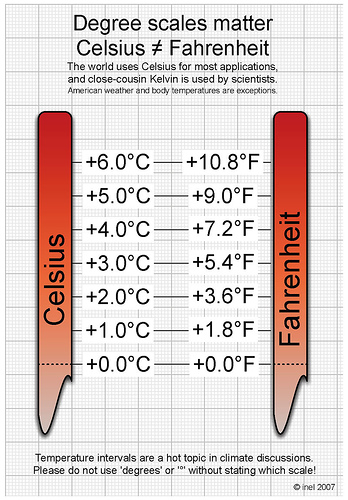# Celsius and Fahrenheit Conversions Made SimpleDo you want to know how many degrees Fahrenheit is 20 degrees Celsius???

Find it out applying the formula below and practicing your addition, subtration and multiplication. Now you can try to do this with the temperatures during the whole week (month, year,...).

Converting Celsius to Fahrenheit:

1. Ask students to think of a temperature in Celsius.
2. Have them add 40 to that number.
3. Multiply the new number by 1.8.
4. Subtract 40.

See example below:

0° Celsius = ?° Fahrenheit
Step #1: 0°C + 40 = 40.
Step #2: 40 x 1.8 = 72.
Step #3: 72 - 40 = 32°F.

# Converting Fahrenheit to Celsius:

1. Think of a temperature in Fahrenheit.
2. Add 40 to that number.
3. Multiply the new number by .55555 (a decimal point followed by five 5s).
4. Subtract 40.

See example below:

32° Fahrenheit = ?° Celsius
Step #1: 32°F + 40 = 72.
Step #2: 72 x .55555 = 39.9996 (round to nearest whole number).
Step #3: 40 - 40 = 0°C.